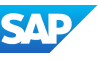New update is available. Click here to update.
Topics

Easy0/40
Average time to solve is 15m+36 more companies

## Problem statement

Example:

``````Input:
2 4 5 -1

Output:
5 4 2 -1

Explanation: 2->4->5 is the initial linked list. If we reverse this, we get 5->4->2.
``````
Detailed explanation ( Input/output format, Notes, Images )
Sample Input 1 :
``````1 2 4 -1
``````
Sample Output 1 :
``````4 2 1 -1
``````
Explanation for Sample Input 1 :
``````1->2->4 is the initial linked list. If we reverse this, we get 4->2->1.
``````
Sample Input 2 :
``````1
1 1 1 -1
``````
Sample Output 2 :
``````1 1 1 -1
``````
Constraints :
``````1 <= 'N' <= 10^4
0 <= 'data' <= 10^9

Where 'N' is the number of nodes in the linked list.

Time Limit: 1 sec
``````Console